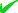News By Tag
Industry News
News By Location

Country(s)

Industry News

May 2022
FrThWeTuMoSuSa
20191817161514

# Guidelines to Work Out the Total Loads on a Column and Related Footing

By: GTR Tech

KOLKATTA, India - Aug. 10, 2020 - PRLog -- In this useful civil engineering article, you will be familiar with the manual method for computing the loads for column and footings design.

• Self weight of the column x Number of floors
• Self weight of beams per running meter
• Load of walls per running meter

The columns are susceptible to bending moments which should be included in the final design.

The structural loading calculations are usually performed on the basis of the following fundamental assumptions.

For Columns

Self weight of Concrete approximately remains 2400 kg per cubic meter, that is equal to 240 kN. Self weight of Steel is approximately 8000 kg per cubic meter. If a large column is considered with size of 230 mm x 600 mm with 1% steel and 3 meters standard height, the self weight of column remains approximately 1000 kg per floor, that is equal to 10 kN. Here, self weight of column is taken as 10 to 15 kN per floor.

For Beams

The calculations are same as above. Suppose, each meter of beam  contains the dimensions of 230 mm x 450 mm exclusive of slab thickness. Therefore, the self weight remains approximately 2.5 kN per running meter.

For Walls

Density of bricks differs among 1500 to 2000 kg per cubic meter. For a 6″ thick wall with 3 meter height and 1 meter length, the load per running meter is calculated to be equivalent to 0.150 x 1 x 3 x 2000 = 900 kg that is equal to 9 kN/meter. This method is useful for measuring the load per running meter for different types of bricks.

For autoclaved, aerated concrete blocks similar to Aerocon or Siporex, the weight per cubic meter remains among 550 to 700 kg per cubic meter. When these blocks are utilized for construction, the wall loads per running meter remains as low as 4 kN/meter, and consequently the construction cost is reduced considerably.

For Slab

Suppose, the thickness of the slab (http://www.constructionnews.co.in/beam-slab-construction-detail.html) is 125 mm. Now each square meter of slab contains a self weight of 0.125 x 1 x 2400 = 300 kg that is equal to 3 kN. Suppose, the finishing load is 1 kN per meter and superimposed live load is 2 kN per meter. Therefore, the slab load can be measured as approximately 6 to 7 kN per square meter.

Factor of Safety

Finally, when the complete load on a column is calculated, consider the factor of safety. For IS 456:2000, the factor of safety should be 1.5.

Contact
Rajib Dey
***@gmail.com
End
Email Tags : ***@gmail.com : Engineering : Construction : Kolkatta - West Bengal - India : Reports
Account Phone NumberDisclaimer     Report Abuse